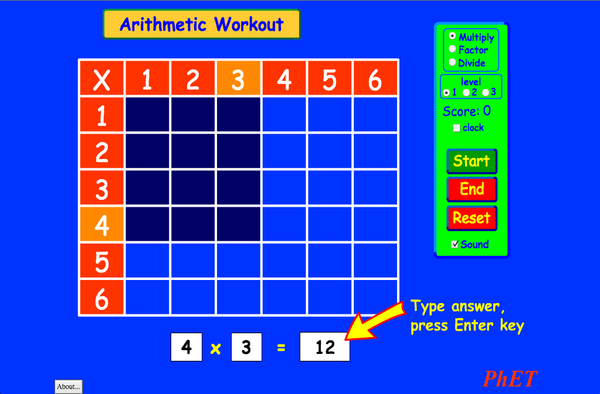# 計算ダウンロード組み込む 閉じる このシミュレーションの実行可能なコピーを組み込む このシミュレーションの実行可能なコピーを組み込むためには、このHTMLコードをお使いください。HTMLコード内の"width"と"height"の属性を変更することによって、組み込まれたシミュレーションの幅と高さを調整できます。 シミュレーション起動用の画像を組み込む
クリックして実行
「クリックして実行」と書かれたスクリーンショットを表示するためには、このHTMLコードをお使いください。 掛け算 PhETの支援者あなたの様な教育者の方々Back to HTML5 Version

• 掛け算

### 学習目標例

• 掛け算の九九を知ることが、どのように掛け算や因数分解や割り算を理解するために役に立つか説明します。
• 掛け算、因数分解、割り算の精度が高まります。

### Standards Alignment

#### Common Core - Math

3.OA.A.1
Interpret products of whole numbers, e.g., interpret 5 × 7 as the total number of objects in 5 groups of 7 objects each. For example, describe a context in which a total number of objects can be expressed as 5 × 7.
3.OA.A.2
Interpret whole-number quotients of whole numbers, e.g., interpret 56 ÷ 8 as the number of objects in each share when 56 objects are partitioned equally into 8 shares, or as a number of shares when 56 objects are partitioned into equal shares of 8 objects each. For example, describe a context in which a number of shares or a number of groups can be expressed as 56 ÷ 8.
3.OA.A.4
Determine the unknown whole number in a multiplication or division equation relating three whole numbers. For example, determine the unknown number that makes the equation true in each of the equations 8 × ? = 48, 5 = _ ÷ 3, 6 × 6 = ?
3.OA.B.5
Apply properties of operations as strategies to multiply and divide.2 Examples: If 6 × 4 = 24 is known, then 4 × 6 = 24 is also known. (Commutative property of multiplication.) 3 × 5 × 2 can be found by 3 × 5 = 15, then 15 × 2 = 30, or by 5 × 2 = 10, then 3 × 10 = 30. (Associative property of multiplication.) Knowing that 8 × 5 = 40 and 8 × 2 = 16, one can find 8 × 7 as 8 × (5 + 2) = (8 × 5) + (8 × 2) = 40 + 16 = 56. (Distributive property.)
3.OA.B.6
Understand division as an unknown-factor problem. For example, find 32 ÷ 8 by finding the number that makes 32 when multiplied by 8.
3.OA.C.7
Fluently multiply and divide within 100, using strategies such as the relationship between multiplication and division (e.g., knowing that 8 × 5 = 40, one knows 40 ÷ 5 = 8) or properties of operations. By the end of Grade 3, know from memory all products of two one-digit numbers.
3.OA.D.9
Identify arithmetic patterns (including patterns in the addition table or multiplication table), and explain them using properties of operations. For example, observe that 4 times a number is always even, and explain why 4 times a number can be decomposed into two equal addends.
Version 2.03

### Teacher Tipsインストラクター用ガイド（pdf）には、PhETチームが作成したヒント集が含まれています。 ( PDF ).

### Teacher-Submitted Activities

Juego de Aritmética (Basado en Indagación)Trish Loeblein, traducido por Diana López 中学

Guided

How do PhET simulations fit in my middle school program?Sarah Borenstein 中学 その他 生物学

ARITHMETIC_DIVISON Arlete Cabral 小学 実験 数学
OBJETO DE APRENDIZAGEM: ARITHMETIC Arlete Moura de Oliveira Cabral e Maria Cleide da Silva Barroso 小学 Guided 数学
Operações Aritméticas Fundamentais Georgyana Cidrão; Francisco Régis Vieira Alves 小学 実験
Guided

MS and HS TEK to Sim Alignment Elyse Zimmer 中学

その他 化学

Math facts - Using the Multiplication Chart Tapan Sarkar 中学 実験 数学
Back to the Basics Nyetta Abernathy 小学

Discovering divisibility rules Stacy Larson 中学

곱셈 나눗셈 연습 SIM 사용설명서 이화국(Wha Kuk Lee) 小学

アフリカーンス語 All AfrikaansRekenkunde
アムハラ語 All Amharicየሒሳብ ስሌቶች
アラビア語 All العربيةarithmetic
アラビア語（サウジアラビア） All العربية (السعودية)arithmetic
アルバニア語 All shqipAritmetika
イタリア語 All italianotabelline
インドネシア語 All Bahasa IndonesiaAritmatika
エストニア語 All EestiAritmeetika
オランダ語 All NederlandsRekenen
カザフ語 All KazakhАрифметика
ギリシャ語 All ΕλληνικάΑριθμητικός
グルジア語 All ქართულიარითმეტიკა
クロアチア語 All hrvatskiAritmetika
スウェーデン語 All svenskaMatteräkning
スペイン語 All españolAritmética
スペイン語（ペルー） All español (Perú)Aritmética
スペイン語（メキシコ） All español (México)Aritmética
スロバキア語 All SlovenčinaAritmetika
スワヒリ語 All SwahiliHesabu
セルビア語 All СрпскиАРИТМЕТИКА
デンマーク語 All DanskAritmetisk
ドイツ語 All DeutschRechnen
トルクメン語 All TurkmenArifmetika
トルコ語 All TürkçeAritmetik
バスク語 All EuskaraAritmetika
ハンガリー語 All magyarSzorzótábla játék
ブラジルポルトガル語 All português (Brasil)Aritmética
フランス語 All françaisArithmétique
ベトナム語 All Tiếng ViệtSố học
ヘブライ語 All עבריתחשבון
ペルシャ語 All فارسیحساب
ボスニア語 All BosanskiARITMETIKA
ポーランド語 All polskiArytmetyka
マケドニア語 All македонскиАРИТМЕТИКА
マラッタ語 All मराठीअंकगणित
ラトビア語 All LatviešuAritmētika
ルーマニア語 All românăAritmetica
ロシア語 All русскийАрифметика

Windows Macintosh Linux
Microsoft Windows
XP/Vista/7/8.1/10
Macromedia Flash 9 or later
OS X 10.9.5 or later
Macromedia Flash 9 or later
Macromedia Flash 9 or later
デザインチーム サードパーティのライブラリ 謝辞